﻿ On the Origin of the CMB RadiationPublications are Open
Access in this journal
Article Versions
Export Article
• Normal Style
• MLA Style
• APA Style
• Chicago Style
Open Access Peer-reviewed

### On the Origin of the CMB Radiation

Jarl-Thure Eriksson
International Journal of Physics. 2021, 9(5), 240-244. DOI: 10.12691/ijp-9-5-3
Received August 03, 2021; Revised August 14, 2021; Accepted August 23, 2021

### Abstract

According to the standard model the CMB radiation is a relict of the Big Bang. Even if the temperature has varied over the years, we now have an accurate figure, 2,72548 K. The radiation exhibits an almost perfect black body spectrum, which causes some confusion, the number of photons required does not match the number available at a distant moment in the past. Several scattering mechanisms have been suggested to give the photon number the necessary gain. It is assumed that the last scattering at ca 380.000 yr after the big bang has left the radiation pattern we now observe. Some notable physicists have expressed doubts about the last scattering and emphasized that the photons originate from positron-electron (e+-e-) annihilations and that scattering would not preserve the black body spectrum. Based on known laws of physics a theory, CBU for the Continuously Breeding Universe, has been developed. The theory incorporates important ideas from the past. The universe is a complex emerging system, which starts from the single quantum fluctuation of a positron-electron pair. Expansion is driven by the emersion of new pairs. Typically, the gravitational parameter G is inversely proportional to the radius. The theory predicts correctly the radius of the observable universe, the Hubble parameter, the energy content and gives a plausible explanation to dark energy. The CBU theory postulates that the primordial universe undergoes a transition from a black hole to a photon filled universe. After the transition one half of the energy is bound to a great multitude of ‘small’ black holes, while the other half propagate as the CMB radiation in the free space. It is presumed that the CMB photons are due to e+-e- annihilations. The frequency-energy (hf) of the photons decreases according to ac2, where ac is the scale factor of the transition. As a characteristic feature the CMB photons are pairwise entangled and in a state of superposition. If we assume that photons in a superposition cannot give off energy, they compensate the hf loss by increasing the number of photons. As they move in all directions the gain will be 1/ac3. In addition, when the photons enter the observable universe at afl (first light), the Doppler effect lengthens the wavelength, whereby the 1-dimensional photon ray to be observed on Earth gets a number gain of 1/afl in compensation, the total gain being 1/afl ac3. The gain effect and the half-energy at the CMB transition result in an energy density of 4,173·10-14 J/m3, equal to αBT04, where αB is the Stefan-Boltzmann black body energy density constant.

### 1. Introduction

Over the years some renowned astronomers and astrophysicists have been sceptic about the big bang and the CMB as its fingerprint, among those were Fred Hoyle, Jayant Narlikar, Halton Arp and Nobelist Hannes Alfvén, cf. Wikipedia “Non-standard cosmology”. One recent time doubter is Hans-Jörg Fahr, a professor of astronomy at the University of Bonn. According to Fahr “there is a substantial lack of theoretical understanding of the CMB phenomenon”, 1.

In a 2012 paper Rishi Khatri and Rashid Sunyaev of the Max Planck Institute presented a comprehensive survey entitled “Creation of the CMB spectrum: precise analytic solutions for the blackbody photosphere”, 2. The objective was to show that the different mechanisms of double Compton, Compton scattering on thermal electrons (the Sunyaev-Zeldovich effect, 3) and bremsstrahlung are capable to provide the necessary number gain of the CMB photons to fit the present spectrum. Recently Sunyaev, however, at a Princeton Physics Group meeting, 4, expressed his hesitancy by saying: “There is an obvious question ‘How and at which redshift the observed, practically ideal, black body spectrum of Cosmic Microwave Background Radiation was created’?”

The renowned physicist Paul Dirac presented his Large Number Hypothesis (LDH) in 1937. In the early 70ies he was still convinced that there is a ‘continuous creation’ and that G varies with time, 5, 6. E. P. Tryon suggested in a Nature article in 1973 that the universe was initiated by a positron-electron quantum fluctuation, 7. In 1994 Alan Guth, the physicist behind the inflation theory, proposed that the total energy of the universe is zero. Matter and radiation provide the positive part, while the potential energy of gravity forms the negative part, 8.

The article is a continuation of a comprehensive study aiming at showing that the Dirac LNH has an anchorage in general relativity and leads to a description of the universe in accordance with recent satellite data. The CBU theory (Continuously Breeding Universe) was developed by the author in ref. 9, together with a Coriolis explanation of galaxy dynamics. The theory is based on the assumptions mentioned above.

Recently it was shown that the Einstein Field Equation can be formulated in the original form Gμν=Tμν wherein Gμν is the Einstein tensor and Tμν is the energy-momentum tensor, 10. The momentum change, expansion pressure and gravitational parameter G must be included in the density and pressure terms of the Tμν-tensor in order to find the correct formulation.

Quantum mechanics is vital for the explanation of the universe expansion. A solution to the Schrödinger equation of the initial event has a crucial impact on the excitation of real positron-electron pairs from the virtual ground state foam. According to the theory the energy is confined to matter and radiation, neither dark energy nor dark matter is required. However, a certain part of the quantum foam, from which real matter emerges, may be considered as virtual dark energy, which in an accumulated form corresponds to the dark energy of the ΛCDM theory.

In the present article it is shown that the most plausible explanation to the CMB radiation and its black body spectrum requires the annihilation of positrons and electrons plus the successive increase of positive energy in the form of matter balanced by the negative gravitational potential energy. The CBU theory offers a solution.

### 2. The Universe, an Odd Black Hole

A closed universe should be considered as a black hole, from where light does not escape. The energy content corresponds to an estimated radius ru, smaller than the Schwarzschild radius rs: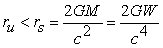(1)

where G is the gravitational parameter, c the speed of light, M and W are the mass and the energy respectively.

A classical black hole has an event horizon at rs. Instead of having a certain distribution of radiation and matter the space inside the black hole curves into a singularity, into which a tremendous attraction force drives everything inside the event horizon. The present universe is an odd black hole, because there is no singularity, only a part of the energy is concentrated into a multitude of ‘small’ black holes in the galaxy centers, while the rest is distributed among stars, planets, interstellar particles and radiation. The average energy density is very small.

The universe differs in another respect from the classical black hole, it expands with a velocity close to the speed of light. It has been shown that the expansion is accelerating, 11. In Appendix A the acceleration equation is presented and further used to determine the Hubble parameter and the radius-time relation, 10.

As observers inside this odd black hole, we experience an outer border of light penetration, the inner photon sphere of a black hole. The radius is obtained by assuming the equivalent mass of a photon to be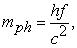(2)

where h is the Planck constant and f the photon frequency. In accordance with the law of gravitation we equate mph with the potential energy of the gravitational field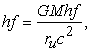from which we have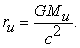(3)

Here Mu and ru stand for the total mass and the outer radius of the universe. As seen the radius is half that of the Schwarzschild radius. The result correlates with theories presented by Paul Dirac, 6, Dennis Sciama, 12, and C. Brans & R. Dicke, 13.

We postulate that the radius r of the observable universe is half of the overall radius ru. The reasoning in the next sections will show that this is a plausible procedure. Given that Wu = Muc2 we have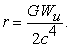(4)

### 3. The Emerging Universe

According to our doctrine the universe emerged from a positron-electron quantum fluctuation and continues to expand due to the inflow of new e+-e- pairs. Initially the inflow is very intense as the extremely large gravitational parameter G magnifies the Planck length and Planck time, thereby widening the Heisenberg uncertainty window.

In ref. 10 it was shown that the curvature radius equals the radius of the observable universe and that the radius ri of the initial universe is obtained from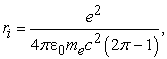(5)

where e and me are the electron charge and mass respectively, 0 is the vacuum permittivity. We have that ri = 0,53380·10-15m.

It has also been established, 10, that the following equation holds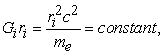(6)

or more generally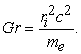(7)

For G = G0 = 6,674·10-11 m3/kgs2, the present gravitational parameter (Newton’s constant), we have that r0 = 4,206·1026 m, the radius of the observable universe, slightly smaller than the standard model 4,4·1026 m.

From eqs (4) and (7) follows that the total energy of the universe is a multiple of the number of e+-e- fluctuations equal to (r/ri)2. We have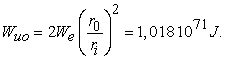(8)

Here We = mec2 = 8,1871056·10-14 J. The equation indicates that energy is proportional to r squared.

According to satellite data the critical density is ca. 9·10-27 kg/m3, the relative matter+radiation factor Ωbr = 0,05, 14. Assuming an estimated volume of 2,50·1081 m3 the energy is WuS = ρcr c2ΩbrVu = 1,011·1071 J, a deviation less than 1 %.

The author has defined the relation between the energy and the radius as follows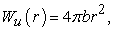(9)

where b = 0.4580·1017 J/m2 is a universal energy surface density constant. b is obtained from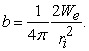(10)

### 4. Redshift in a Strong Gravitational Field

The gravitational parameter G is extremely large in the initial phase, Gi ≈ 5·1031 m3/kgs2. The parameter decreases successively with the expansion. The photons of the Cosmic Microwave Background (CMB) are propagating faster than the expansion and must climb a gravitational upward slope. We write the energy equation for electromagnetic radiation propagating in a gravitational gradient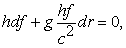(11)

where f is the frequency, g the gravitational acceleration along r. We obtain g from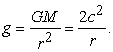(12)

Here we made use of M = 4πbr2/c2 and G=c4/2πbr, cf. eq. (4). Having that f = c/λ, df = -cdλ/λ2 and r = r0a, where a is the scale factor, we write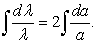(13)

Integration implies that λ is proportional to a2. We deduce that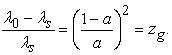(14)

Here zg is the gravitational redshift, λs and λ0 are the wavelengths at the source and at the observer respectively.

As known the cosmological redshift is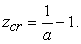(15)

The combined redshift is z = zg + zcr. We have

Let ac stand for the scale factor at the CMB event. For vary small values of the scale factor the frequency of CMB photons decrease approximately according to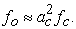(17)

### 5. The Energy Change of the CMB Photon

It is broadly believed that positron-electron annihilation is the primary source of CMB photons, cf. 2, 15. The alternative redshift paradigm of the previous section brings formally the CMB event closer to the present.

The energy density of the black body radiation we obtain from the classical Stefani-Boltzmann equation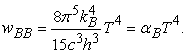(18)

Here αB = 4σSB/c = 7,565723·10-16 J/K4m3 is the density constant, σSB is the Stefani-Boltzmann constant, and kB the Boltzmann constant.

The number density of the photons is obtained from, cf. Wikipedia: Photon Gas,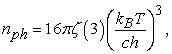(19)

where ζ(3) = 1,202056 is the Riemann zeta-function. By dividing eq. (18) with eq. (19) we obtain an expression for the photon energy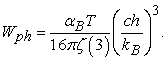(20)

If we assume that the photon energy at the CMB event equals one of the two photons caused by the annihilation, we have Wphc = We. The present time photon energy is then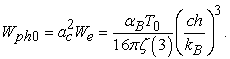(21)

Accordingly, for T0 = 2,72548 K we have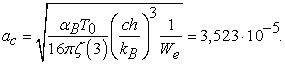(22)

Due to the square root the figure is much smaller than usually suggested, 10-7…10-9.

The present photon energy is Wpho = 1,0164·10-22 J, eq. (21), and the corresponding frequency f0 = Wph0/h = 153,4 GHz, slightly below the Planck black body spectrum optimum frequency of fImax = 160,23 GHz.

### 6. Characteristics of the CMB Photons

Presuming that the CMB photons originate from e+-e- annihilations, the photons are entangled in a state of superposition. They do not follow the same rules as photons from a usual light source, a star, say.

There are divergent opinions about the destiny of the energy lost by light propagating in the expanding universe. Light waves in a gravitational field are part of the system, gaining or loosing potential energy. The CMB photons, however, are different.

It is a well-known fact that the number density of CMB photons, nph0 ≈ 4·108 m-3, is in poor conformity with a value predicted by e+-e- annihilations, the number requires a boost. The state of superposition offers a novel approach. The energy lost as a light wave is compensated by an addition of the numbers of photons, even if the photons in a superposition are not a multitude of distinctive particles.

The expansion advances in all 3D directions, the number of photons increases according to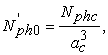(23)

Where Nphc is the number of photons at ac and N’ph0 is the present number due to expansion.

When photons enter the observable universe at a scale factor of afl (first light), they so to speak pass a single slit, a moment continuously changing with expansion, only to be observed at the planet Earth in the Milky Way galaxy after about 14,2 Gyr, cf. App. A. During the passage the relativistic Doppler effect increases the wavelength and thereby lower the photon energy. As they still are in superposition the compensation means an increase of numbers proportional to 1/ afl, a 1-dimensional action because of a specific single ray.

The total number of CNB photons at present is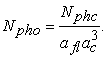(24)

### 7. The Energy Density of the CMB

We postulate that the primordial black hole universe from section 2 undergoes a transition when half of the energy is due to the radiation caused by e+-e- annihilations and the other half is still bond to matter. The superposition state of the photons means that they lack an impact on gravitation. The radius of the universe exceeds the critical Schwarzschild radius, whereby the radiation is liberated into the free space, while matter is divided into a great multitude of ‘small’ black holes, the seed of galaxies to evolve. A simple scheme describes the transition: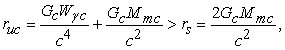(25)

where Gc is the gravitational parameter, Wγc is the radiation energy and Mmc is the mass of all matter at ac.

At the transition we have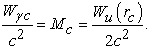(26)

The CMB energy is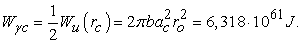(27)

The number of photons is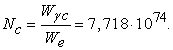(28)

The scale factor for the ‘first light’ moment of the observable universe was determined in ref. 9: afl = 0,01734, a value resembling tfl = 0,255 Gyr, cf. App. A, which is in good conformity with present estimates.

The total number of photons is, eq. (24),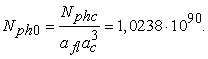The number density is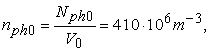(29)

where V0 = 2,493·1081 m3. nph0 is in good agreement with present data.

The energy density of the CMB is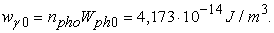(30)

The result is practically identical with wBB0 = αBT04 = 4,1747·10-14 J/m3.

### 8. Conclusion

We have arrived at an almost perfect solution to the CMB problem along three independent paths. Firstly, the universe starts as an odd black hole, wherein fast expansion prevents the formation of a singularity. Quantum fluctuations cause an inflow of positron-electron pairs, some of which annihilate and create the CMB photons. When the energy of the radiation equals the energy of matter there is a transition, whereby matter is concentrated into a multitude of ‘small’ black holes and the radiation is freed.

Secondly, a novel - as compared to the standard model definition - CMB scale factor ac characterizing the liberation event of the black body photons was determined from known photon gas equations. The scale factor is approximately the square root of that usually connected with the annihilation. Despite that, the time tc, 539 kyr, is close to the standard model value of 380 kyr. According to the CBU theory the time dependence of the universe size does not follow the Friedmann 2/3 power law.

Thirdly, photons originating from annihilations are pairwise entangled and in a superposition. The photons are scattered in all 3D directions, they are multiplied to compensate for the energy loss due to the wavelength prolongation caused by the expansion. As a result, the photon number gets a 1/ac3 gain. Similarly, the relativistic Doppler effect causes a 1D multiplication of the photons travelling from the entrance into the observable universe at afl to the present observer, the total gain then being 1/aflac3. The half energy transition at ac and the total gain result in a CMB energy density equal to αBT04.

The photon number gain does not mean that energy is created, but that entangled photons in superposition propagate without losses. The multiplication effect is a scientific proposition, which still requires proof. If true, we come one step closer to a deeper understanding of the dual character of electromagnetic radiation.

### Appendix A

Determination of the Hubble parameter and the time-radius relationship of the expanding universe

The purest form of the classical Einstein field equation is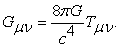(A1)

In ref. 10 it was found that a comprehensive and logic solution based on quantum mechanics leads to an accelerated expansion without a cosmological constant.

The acceleration can be expressed as follows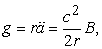(A2)

where B = 0,099153 is a universal constant, or very close to a constant over a wide range of time. g was used for acceleration to prevent confusion with the scale factor.

In order to find the Hubble parameter and the time function of the radius we write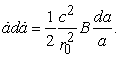(A3)

By integration we obtain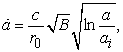(A4)

where ai = ri/r0 = 1,26814·10-42. As a/ai = r/ri we write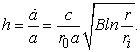(A5)

This is the Hubble parameter, presently h0 = 2,204·10-18 s-1 = 68,02 km/sMpc. The value is close to the currently best estimate 67,7 km/sMpc.

In the next step we substitute u = ln(a/ai) into eq. (A5)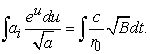(A6)

The integral on the left-hand side has an error function solution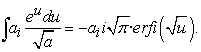(A7)

The real part solution is obtained by using the Dawson integral function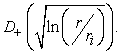Time as a function of radius r is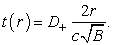(A8)

D+ is directly obtainable from Wolfram Alpha (Dawson(x)): D+ = 0,0511743 for a = 1.

The age of the universe at r = r0 is t0 = 4,560·1017 s = 14,45 Gyr, slightly higher than the official estimate of 13,8 Gyr, discrepancy less than 5 %.

The events of the CMB and the first light occured as follows:

CMB, D+c = 0.0541663, tCMB = 1,701·1013 s = 539.000 yr, slightly later than the standard model 379.000 yr.

First light, D+fl =0,052299, tfl = 8,034·1015 s = 0,255 Gyr

### References

  Fahr, H.-J., Sokaliwska, M., Remaining problems in interpretation of the Cosmic Microwave Background, Physics Research International. (2015). Article ID 503106, 2015. In article View Article  Khatri, R., Sunyaev, R. A., Creation of the CMB spectrum: precise analytic solutions for the blackbody photosphere, arXiv:1203.2601v2 [astro-ph.CO], 2012. In article View Article  Sunyaev, R. A., Induced Compton scattering by thermal electrons and the low-frequency spectrum of radio sources, Soviet Astronomy 15 (2), 244-252, 1971. In article  Sunyaev, R. A., Two Important milestones in the history of the universe: The last scattering surface, the black body photosphere of the universe and distortions of the CMB spectrum, Physics Group Meeting on May 15, 2019, Institute of Advanced Studies, Princeton University. In article  Dirac, P. A. M., The cosmological constants, Nature 139, 323, 1937. In article View Article  Dirac, P. A. M., Cosmological models and the Large Number hypothesis. Proc. R. Soc. Lond. A., 338, pp. 439-446, 1974. In article View Article  Tryon, E. P., Is the universe a vacuum fluctuation. Nature, 247, pp. 396-397. 1973. In article View Article  Guth, A., The Inflationary Universe: The quest for a new theory of cosmic origins. Perseus Books, 1997. In article View Article  Eriksson, J.-T., A modified model of the universe shows how acceleration changes galaxy dynamics. International Journal of Physics, Vol 6, No. 2, pp. 38-46, 2018. In article  Eriksson, J.-T., Quantum fluctuations and variable G return Einstein’s field equation to its original formulation, 9 (3), 169-177, 2021. In article View Article  Perlmutter, S., Supernovae, Dark Energy, and the Accelerating Universe, Physics Today, April 2003. In article View Article  Sciama, D. W., On the origin of inertia. Monthly Notices of the Royal Astronomical Society, 113, 34-42, 1952. In article View Article  Brans, C., Dicke, R. H., Mach's principle and a relativistic theory of gravitation. Physical Review, 124, 3, pp. 925-935, 1961. In article View Article  Tanabashi, M., et al., Astrophysical constants and parameters, Phys.Rev. D 98, 2019 update. In article  Lineweaver, C. H., Inflation and the cosmic microwave background, arXiv: astro-ph/0305179v1, 2003. In articleThis work is licensed under a Creative Commons Attribution 4.0 International License. To view a copy of this license, visit http://creativecommons.org/licenses/by/4.0/